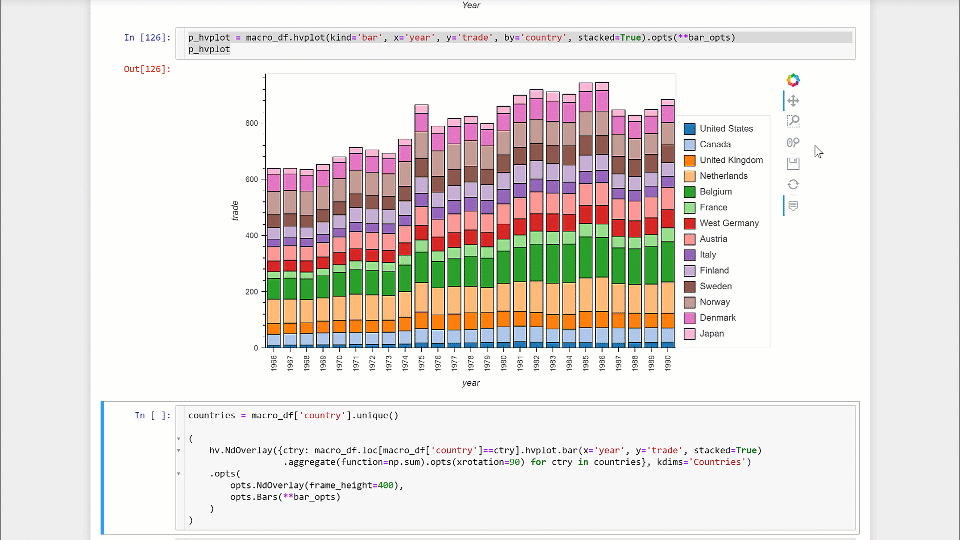# Any way to created working interactive legend for stacked bar chart?

Hi, I am trying to make a stacked bar chart with interactive legend starting with the example in holoviews gallery / bars_economic.

First a some imports and displaying the test-data:

``````import pandas as pd
import holoviews as hv
from holoviews import opts
import hvplot.pandas
import numpy as np
hv.extension('bokeh','matplotlib')

key_dimensions   = [('year', 'Year'), ('country', 'Country')]
value_dimensions = [('unem', 'Unemployment'), ('capmob', 'Capital Mobility'),
macro = hv.Table(macro_df, key_dimensions, value_dimensions)

``````

Trying the holoviews way as in the example with stacked bars. This gives a legend, but it is not interactive.

``````bar_opts = dict(tools=['hover'], frame_width=600, xrotation=90, frame_height=400, color=hv.Cycle('Category20'))

bars = macro.to.bars(['Year', 'Country'], 'Trade', [])

bars.opts(opts.Bars(show_legend=True, legend_position='right', stacked=True, **bar_opts))
``````

Then trying the hvplot path and it leads to pretty much the same results without the added formatting of dimension labels:

``````p_hvplot = macro_df.hvplot(kind='bar', x='year', y='trade', by='country', stacked=True).opts(**bar_opts)
p_hvplot
``````

Finally, I am trying holoviews NdOverlay on a dictionary of bar charts for each country. This gives an interactive legend, but the bars do not stack, i.e. they are overlapping and hiding behind each other.

``````countries = macro_df['country'].unique()

(